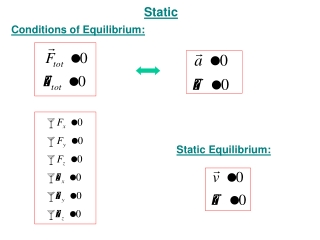DownloadDownload PresentationStatic

# Static

Télécharger la présentation## Static

- - - - - - - - - - - - - - - - - - - - - - - - - - - E N D - - - - - - - - - - - - - - - - - - - - - - - - - - -
##### Presentation Transcript

1. Static Conditions of Equilibrium: Static Equilibrium:

2. Example: Equilibrium and torque 1kg 2kg Not in equilibrium 1m 1m 1kg 2kg In equilibrium 2m 1m

3. Example: You bought a turkey and forgot how many pounds it was. All you have is a tiny kitchen scale that can weigh a maximum of 2 lb. But here’s good trick you can use to get an estimation. How you can do it? Use static equilibrium! When d = 20 cm, the scale reads 2 lb exactly. If L = 1 m, How big is your turkey? f2 d L f1 P Light rod Scale Turkey W

4. Example: On the figure shown below, one end of a uniform road with a weight of 400 N is attached to a wall. The other end of the rod is supported by a wire. What is the tension in the wire? φ =30° φ θ = φ + φ = 60° T θ φ θ mg φ =30° φ Fy Fx T Tcos30° θ φ θ Tsin30° mg

5. Example: A person of mass M climbs a ladder of mass m and length L that leans against a smooth wall. (We can neglect the friction between the wall and the ladder.) The frictional force between the floor and the ladder keeps it from slipping. The angle between the ladder and the wall is φ. Determine how the magnitude of the frictional forcedepends on φ. N2 mg N1 Mg fS φ d C is a good point to use as axis of rotation because then two of the forces will produce zero torque. C

6. Center of gravity (the point where the gravitational force can be considered to act) • The torque due to gravity is the same as if all the mass of the object was concentrated at a point called the center of gravity. • It is the same as the center of mass as long as the gravitational force does not vary among different parts of the object. • It can be found experimentally by suspending an object from different points. r r mg mg torque is not zero torque is zero

7. A. All B. 2 & 3 C. 3 only Example: Three boxes are placed on identical inclines. Friction prevents them from sliding. The boxes are not uniform and their center of mass are indicated by the blue dot in each case. In which cases does the box tip over? 1 2 3 In 3, the CM is not above the base: net ≠ 0

8. Example: A 40-kg plank rests on a roof as shown. A 50-kg flying pig is about to land on the unsupported side. How far from the edge of the roof can the pig land without tipping the plank and itself over? 50 kg 0.5 m x CMpig CMplank 40 kg 2 m 1 m

9. Example: A truck carries a refrigerator of mass M on a horizontal road. The center of mass of the fridge is at height h from the bed of the truck and the width of the fridge is 2d. What is the maximum acceleration aM that the truck can have without tipping the fridge? (Assume the static friction between the truck and the fridge is large enough for the fridge not to slip). CM Mg N 2d CM a h M When the truck has no acceleration (or is at rest), the normal force is right below the CM.

10. CM CM a a Mg Mg N N fS fS When the truck has some acceleration (< aM) to the left, the normal force “moves” to the right to produce enough torque (about the CM) to cancel the torque due to friction (about the CM)! “Moves” = “The pressure on the ground is redistributed” When the fridge is just starting to tip over, the normal acts on the bottom right edge of the fridge. The fridge tips over because the normal force cannot produce a torque larger than Nd.